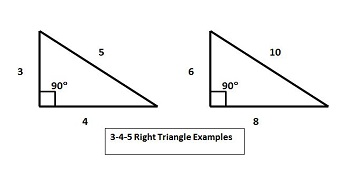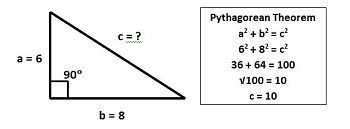# Special Right Triangles: 3-4-5 Triangle

Instructor: Nola Bridgens

Nola has taught elementary school and tutored for four years. She has a bachelor's degree in Elementary Education, a master's degree in Marketing, and is a certified teacher.

After completing this lesson, you will be able to identify and describe the characteristics of a 3-4-5 right triangle and use the Pythagorean Theorem. You will also be able to discuss situations where 3-4-5 right triangles would be useful.

## Characteristics of a 3-4-5 Right Triangle

A right triangle is any triangle with one right angle of 90o. There are several kinds of right triangles, but the 3-4-5 right triangle has special characteristics. The measurements of the sides of a 3-4-5 right triangle are in the ratio 3:4:5. A ratio is the relationship between numbers that shows proportion. As long as the length of the sides of a triangle are proportionate to 3:4:5, it is a 3-4-5 right triangle.

To find other triangles that are proportionate, you can multiply each of the numbers in the ratio by the same number. For example, if we multiply by 2 we get 6-8-10, and if we multiply by 10 we get 30-40-50. Both of these triangles are 3-4-5 right triangles because they maintained the ratio of 3:4:5.If you know the length of all the sides of a triangle, you can determine whether it is a 3-4-5 right triangle simply by checking to see if the ratio of the sides is proportionate to 3:4:5. However, if we do not know the measurements of all the sides, we need to calculate them to see if it is a 3-4-5 right triangle.

## Finding the Unknown Side of a Right Triangle

If you do not know the length of one of the sides of a triangle, don't worry. A famous Greek mathematician named Pythagoras discovered a formula to help us. Named after himself, the Pythagorean Theorem can help us determine the length of an unknown side of a right triangle. The Pythagorean Theorem formula is: a2 + b2 = c2 (c is also known as the hypotenuse). As long as we know the length of two of the sides of a right triangle, we can use this method. Let's look at the example. We know that side a = 6 and side b = 8. If we plug the numbers into our formula, we get:

62 +82 = c2

62 = 36 and 82 = 64

36 + 64 = 100

We now know that c2 = 100.

The square root of 100 is 10, so we know that c = 10.

This triangle has the ratio 6:8:10, which is proportionate to 3:4:5, so it is a 3-4-5 right triangle.## Practical Uses of 3-4-5 Triangles

Now you might be saying to yourself, ''This formula and information on 3-4-5 right triangles will help me in math class, but when will I ever use this in real life?'' That is a great question, and I have the answer for you.

To unlock this lesson you must be a Study.com Member.

### Register to view this lesson

Are you a student or a teacher?